List of public pages created with Protopage

# Home

## Rich sticky notes

Welcome to

MrWilliamsMaths

### Digital Backpack### Term 4 - My Classes

Year 7

Number

Adding, subtracting and multiplying with decimals

Rounding to decimal places

Multiply and divide by powers of 10

Estimation

Using a calculator effectively

Equivalence of fraction/decimal/percentage

Transformations

Reflect shapes over a mirror line

Reflect shapes over an axis and simple equations including y=x

Translate shapes

ASSESSMENT 7 – Middle of term 4

Mappings and Graphs

Substitution

Plot co-ordinates of a linear function

Graph any straight line function

Shape, Space and Measure

Draw nets of 3D shapes

Draw 3D shapes on isometric paper

Use scale to draw models

Construct triangles given two lengths and an angle, two angles and a side or all three sides

ASSESSMENT 8 - End of term 4

Year 8

 Statistics Scatter graphs and correlation Two-way tables Draw and use frequency diagrams Drawing pie charts Enlargements and Scale Drawings Whole number scale factor enlargements Use scale drawings Decimals Multiply and divide decimals Using a calculator correctly Solving problems ASSESSMENT 7 – Middle of Term 4 Equations Construct and solve linear equations Solve equations involving fractions Solve equations such as x2=9 Solve more difficult equations using trial and improvement Straight Line Graphs Substitution in to a formula Plotting co-ordinates Sketching straight line graphs using a table of values Sketching straight line graphs using the gradient-intercept method ASSESSMENT 8 - End of term 4

Year 9
 Simplifying Algebra & indices. Substitution Grade Expanding and Simplifying Brackets C Expanding brackets with higher powers e.g. 2x^2(x^3 + 3y) D Recap simplifying indices C/B Using and simplifying negative and fractional indices B/A/A* Surds Simplifying surds A Multiplying and dividing surds A Expanding brackets with surds A* Using surds in problems such as pythagoras A* Rationalising the denominator A* Formulae Substituting into a formula D Writing formula to express a situation D Changing the subject of a formula A/A* recognising/using/rearrangin important shape formulae ASSESSMENT 7 – Middle of term 4 Averages Calculate the mean/mediand/mode for lists of data D/C Calculate the mean from a table of data D Calculate the mean from grouped data C Calculate the median from grouped data C Compound Measure Know a use formula for speed, distance and time. D/C Know a use formula for volume, density and mass B Rearrange both these formula D/C/B Surface Area and Volume of Prisms Calculate the surface area of prisms C Calculate the volume of prisms C ASSESSMENT 8 - End of term 4

## Photos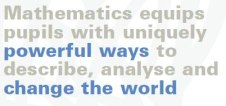### MrWilliamsMaths website for students, parents and teachers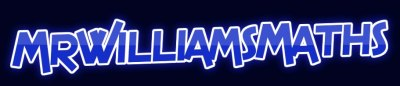### Visit MrWilliamsMaths Blog at www.mrwilliamsmaths.wordpress.com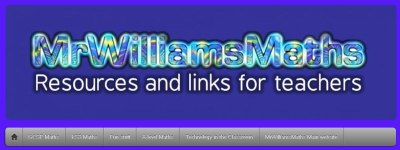# KS3 Information and Resources

## KS3 Level Descriptors

Maths Level 1

To achieve Level One a student must be able to: count, order, read and write numbers up to ten and add/subtract numbers up to ten.  She must be able to describe 2D and 3D shapes using everyday language and say if things are bigger or smaller than each other.  She must also be able to sort objects.

Maths Level 2

To achieve Level Two a student must be able to: know addition/subtraction facts up to 10; use place value to order numbers up to 100; know that addition and subtraction are inverses and choose the right one; mentally solve problems with money or measurements and recognise odd and even numbers.  She must be able to: use mathematical names for common 2D and 3D shapes; describe the number of sides/corners of these shapes; understand that angles measure how much something has turned recognise a right angle and use everyday units to measure length and weight. She must also be able to write information in a list, draw a table and draw a block graph.

Maths Level 3

To achieve Level Three a student must be able to: understand place value up to 1000; make approximations to sums under 1000; use decimal notation as in money; use negative numbers as in temperature; add/subtract 2 digit numbers mentally; add/subtract 3 digit numbers using written methods; know the 2,3,4,5 and 10 times tables; then use them to do division; use simple fractions and recognise when two fractions are equivalent.  She must be able to: group together 2D and 3D shapes according to their properties; understand line symmetry and understand units of length, capacity, weight and time.  She must also be able to read information from a table or list; draw a bar chart; draw a pictogram and get information from a bar chart or pictogram.

Maths Level 4

To achieve Level Four a student must be able to: describe number patterns; find multiples/factors; work out square numbers; use word formulae; use positive co-ordinates; multiply/divide whole numbers by 10 and 100; know times tables up to 10x10; add/subtract decimal numbers like 13.64 and 48.95; order decimal numbers and  check answers.  She will be required to: make 3D models; draw 2D shapes; find perimeters of shapes and find area by counting squares.  She also must draw line graphs; present data clearly; read simple pie charts; draw frequency tables and find the mode and range.

Maths Level 5

To achieve Level Five a student must be able to: multiply/divide whole numbers by 10; 100 and 1000; add/subtract negative numbers; put numbers in order (including negative numbers); add/subtract/multiply/divide decimal numbers; simplify a fraction; work out a fraction/percentage of a number; multiply/divide a 3 digit number by a 2 digit number; use inverse operations of approximation to check answers; use simple formulae and use co-ordinates in all four quadrants.  She will be required to: measure and draw angles to the nearest degree, remember the metric to imperial conversions and use and understand the formula for the area of a rectangle.  She also must: find the mean of discrete data; use the range and an average to compare two sets of data; say what diagrams and graphs show; use the probability scale from 0 to 1 and understand that experiments don't always have the same outcome.

Maths Level 6

To achieve Level Six a student must be able to: work out one number as a fraction/percentage of another; understand that fractions; decimals and percentages can be equivalent to each other; calculate using ratio; add/subtract fractions; find and describe in words the rule for the next term (and nth term) in a linear sequence and solve linear equations and plot a linear graph.  She will be required to: recognise 2D representations of 3D objects; classify quadrilaterals by their properties; find missing angles when two parallel lines are intersected and in polygons; find the area and circumference of a circle; find the volume of cuboids and enlarge a shape.  She also must: be able to work with continuous data; construct pie charts; interpret a scatter diagram; find all the possible outcomes of two experiments and use the fact that the probability of mutually exclusive events adds up to 1.

Maths Level 7

To achieve Level Seven a student must be able to: round to one significant figure, understand what happens when we multiply/divide by numbers between 0 and 1; multiply/divide numbers of any size; understand proportional change; find algebraically the rule for the next term or nth term in a quadratic sequence; expand and simplify quadratics solve simultaneous linear equations and solve linear inequalities.  She will be: required to use Pythagoras' Theorem; calculate lengths, areas and volumes in prisms; enlarge a shape by a fractional scale factor; understand similarity; draw the locus of a moving object; find upper and lowers bounds and use compound measures like speed, distance, time.  She also must: be able to give and test a hypothesis; understand bias; find the modal class and an estimate to the mean, median and range when using grouped data; compare distributions using frequency polygons; draw a line of best fit on a scatter diagram and understand relative frequency.

Maths Level 8

To achieve Level Eight a student must be able to: solve problems involving powers, roots and standard form; solve problems involving repeated proportional change; substitute fractions and decimals into equations and expressions and find the answers; calculate one variable in a formula the others are known; understand that a2-b2=(a+b)(a-b); solve inequalities in two variables; sketch and interpret graphs of quadratic; cubic and reciprocal functions and interpret graphs that model real life situations.  She will be: required to use congruence and mathematical similarity; use sine/cosine/tangent in right angled triangles and distinguish between formulae for perimeter, area and volume by considering dimensions.  She also must: interpret and construct cumulative frequency diagrams; estimate the median and inter-quartile range and calculate the probability of a compound event.

# A-level

## Rich sticky notes

### Important Message from Mr Williams for students

PLEASE CLICK HERE TO GO TO THE YEAR 12 MATHS WEBSITE (It's the same as what's below but it's quicker and easier)

### A LEVEL MATHS WEBSITE

Try this worksheet on equation of a circle

GCSE REVISION EXERCISES - Highly Recommended!

# Number & Algebra

## Photos

### ALGEBRA

Extract from Nayland Maths at http://mathematics.nayland.school.nz/Year_10/y10_Algebra/Y10_Algebra_home.htm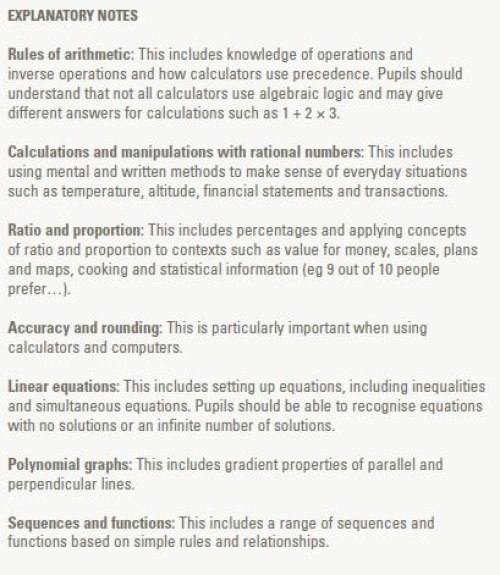### National Curriculum for KS3

Taken from the National Curriculum for Key Stage 3 - http://curriculum.qcda.gov.uk/uploads/QCA-07-3338-p_Maths_3_tcm8-403.pdf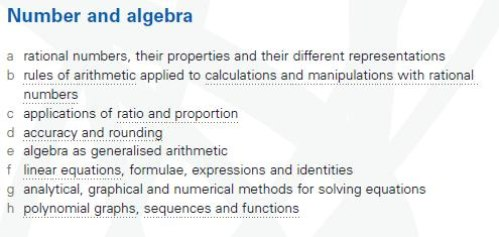# Geometry and Measures

## Rich sticky notes

### Geometry and Measure

4. Geometry and measures

4.1 Geometrical reasoning

4.2 Transformations and coordinates

4.3 Construction and loci

4.4 Measures and mensuration

# Statistics

Get this widget!

## Photos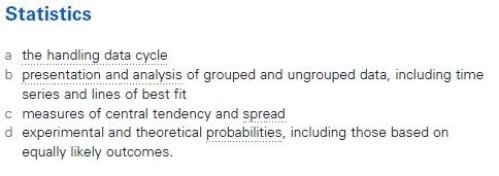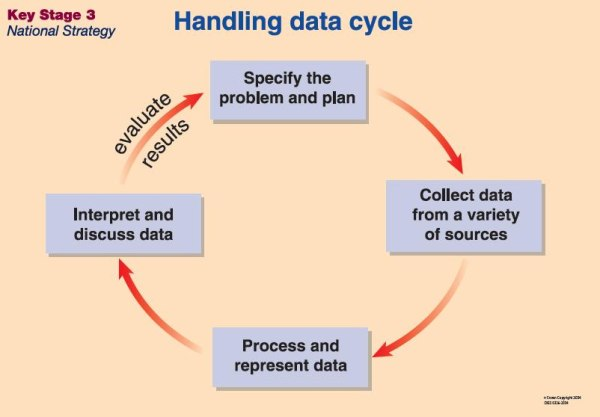# Mathematical Processes & Applications

## Rich sticky notes

### Mathematical Processes & Applications

1. Mathematical processes and applications

1.1 Representing

1.2 Analysing – use mathematical reasoning

1.3 Analysing – use appropriate mathematical procedures

1.4 Interpreting and evaluating

1.5 Communicating and reflecting

### Currency converter

xe.com  Universal Currency Converter ®

### Temperature Conversion

A Widgia Widget# Fun and Games

## Rich sticky notes

### Glitter Memory Game

provided by: widgia.com# TEACHER Links, Tools and Resources

## Rich sticky notes

### Flash files, applets and gizmos

Excellent Flash movies to demonstrate CIRCLE THEOREMS

WisWeb  has some excellent tools, including the fun 24 game.

Flash tools from Milford Haven Schools, including a countdown timer.

### Videos and Multimedia

Video Math Tutor - Brain Teasers

2012-2013